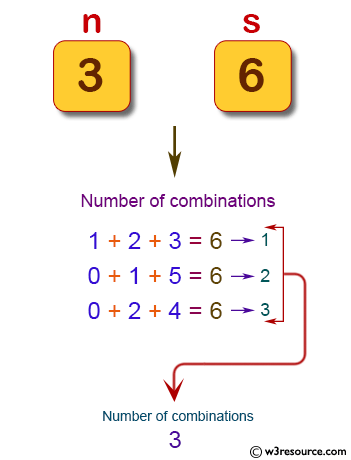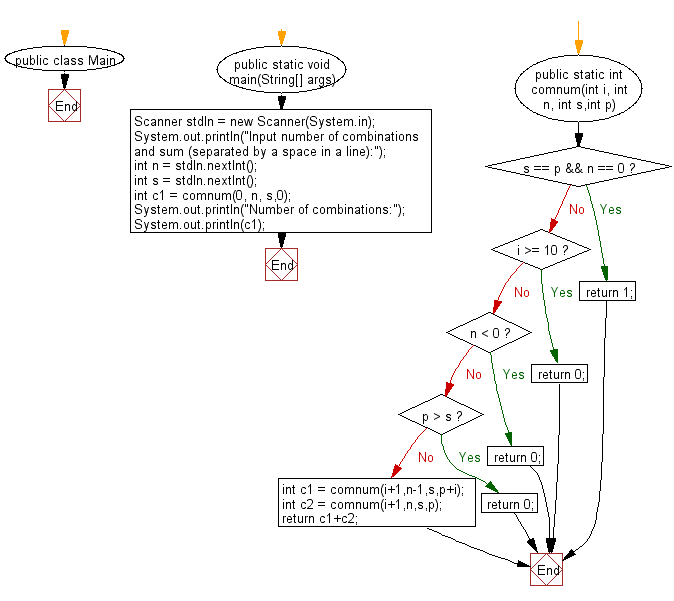# Java Exercises: Reads n digits (given) chosen from 0 to 9 and prints the number of combinations

## Java Basic: Exercise-228 with Solution

Write a Java program that reads n digits (given) chosen from 0 to 9 and prints the number of combinations where the sum of the digits equals to another given number (s). Do not use the same digits in a combination.

For example, the combinations where n = 3 and s = 6 are as follows:
1 + 2 + 3 = 6
0 + 1 + 5 = 6
0 + 2 + 4 = 6

Input:

Two integers as number of combinations and their sum by a single space in a line. Input 0 0 to exit.

Pictorial Presentation:Sample Solution:

Java Code:

`````` import java.util.*;
public class Main {
public static void main(String[] args) {
Scanner stdIn = new Scanner(System.in);
System.out.println("Input number of combinations and sum (separated by a space in a line):");
int n = stdIn.nextInt();
int s = stdIn.nextInt();
int c1 = comnum(0, n, s,0);
System.out.println("Number of combinations:");
System.out.println(c1);
}
public static int comnum(int i, int n, int s,int p) {
if(s == p && n == 0) {
return 1;
}
if(i >= 10) {
return 0;
}
if(n < 0) {
return 0;
}

if(p > s) {
return 0;
}
int c1 = comnum(i+1,n-1,s,p+i);
int c2 = comnum(i+1,n,s,p);
return c1+c2;
}
}
```
```

Sample Output:

```Input number of combinations and sum (separated by a space in a line):
3 6
Number of combinations:
3
```

Flowchart:Java Code Editor:

What is the difficulty level of this exercise?

﻿

## Java: Tips of the Day

Parsing dates:

```import java.io.*;
import java.util.*;
import java.text.*;

String s = "2001/09/23 14:39";

SimpleDateFormat formatter = new SimpleDateFormat ("yyyy/MM/dd H:mm");
Date d = formatter.parse(s, new ParsePosition(0));
```